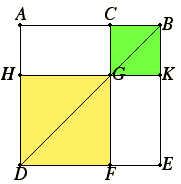# Proposition 4

If a straight line is cut at random, then the square on the whole equals the sum of the squares on the segments plus twice the rectangle contained by the segments.

Let the straight line AB be cut at random at C.

I say that the square on AB equals the sum of the squares on AC and CB plus twice the rectangle AC by CB.Describe the square ADEB on AB. Join BD. Draw CF through C parallel to either AD or EB, and draw HK through G parallel to either AB or DE.

I.29

Then, since CF is parallel to AD, and BD falls on them, the exterior angle CGB equals the interior and opposite angle ADB.

But the angle ADB equals the angle ABD, since the side BA also equals AD. Therefore the angle CGB also equals the angle GBC, so that the side BC also equals the side CG.

I.34

But CB equals GK, and CG to KB. Therefore GK also equals KB. Therefore CGKB is equilateral.

I say next that it is also right-angled.

I.29

Since CG is parallel to BK, the sum of the angles KBC and GCB equals two right angles.

I.34

But the angle KBC is right. Therefore the angle BCG is also right, so that the opposite angles CGK and GKB are also right.

Therefore CGKB is right-angled, and it was also proved equilateral, therefore it is a square, and it is described on CB.

I.34

For the same reason HF is also a square, and it is described on HG, that is AC. Therefore the squares HF and KC are the squares on AC and CB.

I.43

Now, since AG equals GE, and AG is the rectangle AC by CB, for GC equals CB, therefore GE also equals the rectangle AC by CB. Therefore the sum of AG and GE equals twice the rectangle AC by CB.

But the squares HF and CK are also the squares on AC and CB, therefore the sum of the four figures HF, CK, AG, and GE equals the sum of the squares on AC and CB plus twice the rectangle AC by CB.

But HF, CK, AG, and GE are the whole ADEB, which is the square on AB.

Therefore the square on AB equals the the sum of the squares on AC and CB plus twice the rectangle AC by CB.

Therefore if a straight line is cut at random, the square on the whole equals the squares on the segments plus twice the rectangle contained by the segments.

Q.E.D.

## Guide

The statement of the proposition can be interpreted in modern notation as saying that if x = y + z, then x2 = y2 + z2 + 2yz. More simply, as an identity, it says

(y + z)2 = y2 + z2 + 2yz.

#### Use of this proposition

This is one of the more frequently used propositions of Book II. It is used in II.12 later in this book, frequently in BookX, and in XIII.2. Also, the analogous statement for numbers is used in IX15,